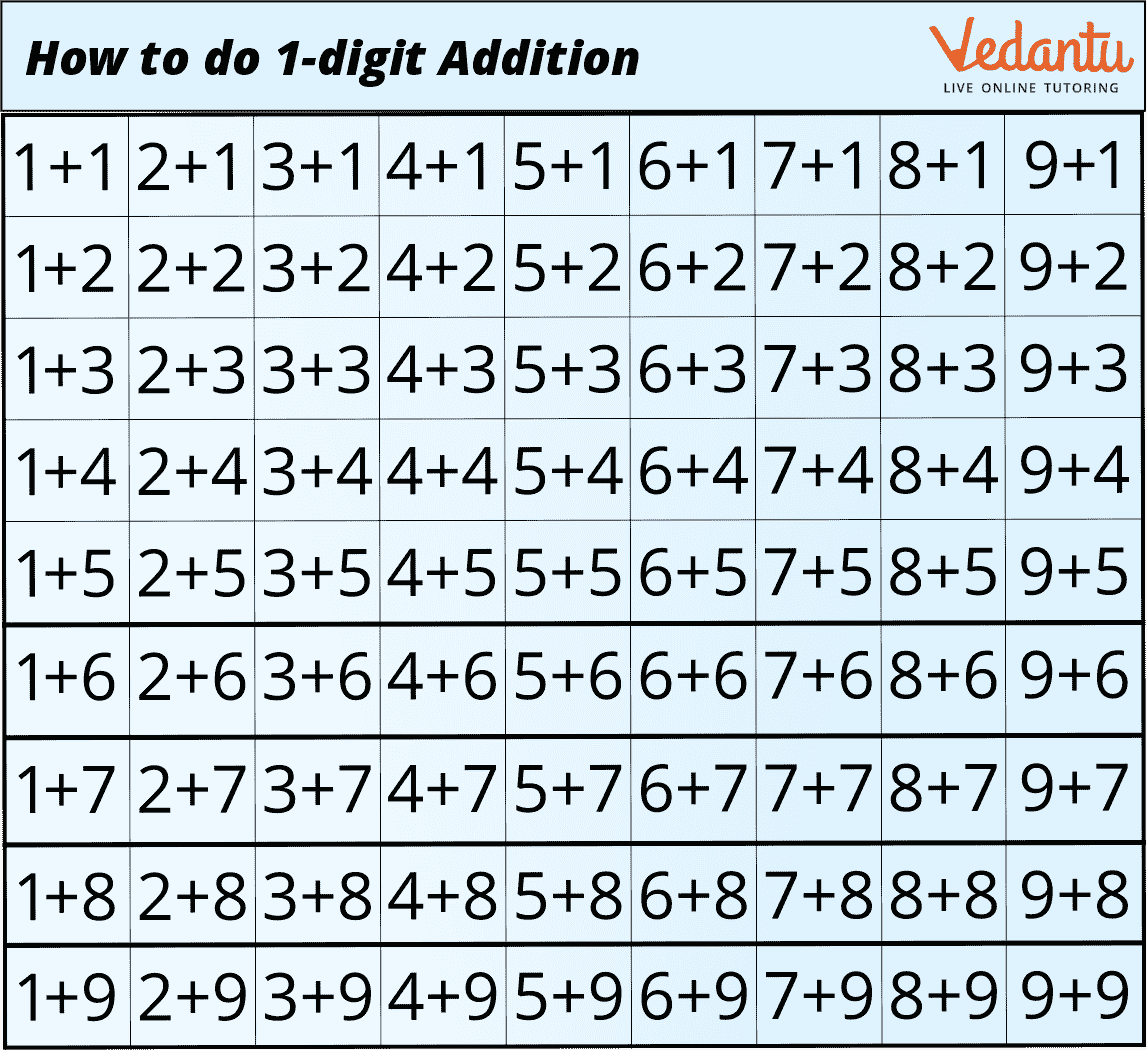Courses
Courses for Kids
Free study material
Free LIVE classes
More

# Addition Facts for Class 1## Introduction

One of the arithmetic operations in mathematics is addition. You must have added at least once before. For example, your parent may offer you an apple in the morning and another apple in the evening. You have eaten a total of 2 apples in one day. How can one get the total? The only way to do this is by adding. As a result, you must add 1 to 1 to obtain the amount.

The addition is not just a process of adding numbers. It is also a foundation for numerous math operations, ranging from simple concepts like “two plus three equals five” to more complex topics like algebraic expressions.

One addition fact is that addition can be defined as the sum of two or more addends.

Example: 2 + 3 = 5

In addition, the place value of the numbers is given more importance. Here, the place value is expressed as ones and tens. To add numbers, we need to arrange them column-wise according to their place values. Each digit is individually added. The answer is known as their total, and they are known as addends.

There are two methods for adding two digits. The first two are the "without regrouping" approach and the "regrouping" method, both referred to as carrying forward.

The sums from 1 + 1 to 9 + 9 are addition facts. Children must learn these 81 sums since they are the foundation for the rest of elementary mathematics. The full list of addition facts is provided below:• The addition of 1-digit numbers can be done horizontally.

Example : 2+3 =5

• The addition of zero to any number does not change the sum.

Example : 5 + 0= 5

• If we add one to any number, it gives the after that number as a sum.

## Solved Numericals:

Example 1: Find out the missing numbers -

1. 1 + 5 = _

2. 4 + _ = 7

3. _ + 3 = 3

Ans: a. 6

b. 3

c. 0

Example 2: Solve the following sums and match them with the correct answer.

Column A Column B

1. 4 + 5 4

2. 6 + 2 7

3. 3 + 1 9

4. 5 + 2 8

Ans: a . 4 + 5 = 9

b . 6 + 2 = 8

c . 3 + 1 = 4

d . 5 + 2 = 7

Example 3: Find the sum of the following numbers -

1. 2 + 7 =

2. 1 + 4 =

3. 6 + 3 =

4. 1 + 0 =

Ans: a. 9

b. 5

c. 9

d. 1

Example 4: Fill in the missing numbers -

1. 6 + _ = 7

2. _ + 3 = 9

3. 7 + _ = 0

4. 1 + _ = 8

Ans: a. 1

b. 6

c. 0

d. 7

## Conclusion:

This article taught us about adding 1 - digit numbers with solved numerals. Adding numbers is a fundamental mathematical process combining two or more numerical values. This operation is used in our daily lives; some simple examples include calculating money, calculating time, counting students in a class, and so on.

Last updated date: 19th Sep 2023
Total views: 77.7k
Views today: 1.77k

## FAQs on Addition Facts for Class 1

1. What is the significance of addition?

Adding numbers is a fundamental mathematical concept required for even the most basic problems in our daily lives. One of the most common uses is when working with money, such as adding bills and receipts.

2. What is the difference between addition with and without regrouping?

When the sum of the digits in each place value column is less than or equal to 9, it is considered to be added without regrouping. When the sum of the digits in at least one of the place value columns is greater than 9, this is considered an addition with regrouping.

3. Why is it important to add math?

Additional mathematics is an extremely beneficial learning experience for students who have mastered lower secondary school mathematics. It helps you develop deeper mathematical competency and opens up many opportunities for further education.